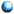Date: 2003/09/25 15:00-17:00Place: Lecture Hall(Knowledge Science)Name: Kaoru KUROSAWA
$B!!!!!!!!!!(BDepartment of Computer and Information Sciences,$B!!(BIbaraki UniversityTitle: New provably secure PKC and MAC schemesContents:

 In the first part, we study some RSA-based semantically secure encryption schemes (IND-CPA) in the standard model. We first derive the exactly tight one-wayness of Rabin-Paillier encryption scheme which assumes that factoring Blum integers is hard. We next propose the first IND-CPA scheme whose one-wayness is equivalent to factoring {\it general} $n=pq$. In the second part, we present One-key CBC MAC (OMAC) and prove its security for arbitrary length messages. OMAC takes only one key, $K$ ($k$ bits) of a block cipher $E$. Previously, XCBC requires three keys, $(k+2n)$ bits in total, and TMAC requires two keys, $(k+n)$ bits in total, where $n$ denotes the block length of $E$.

[back]